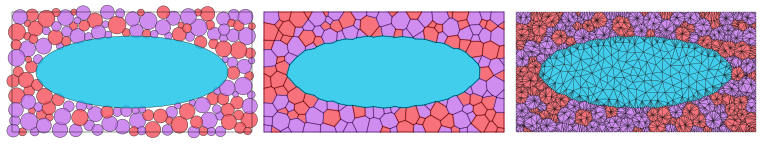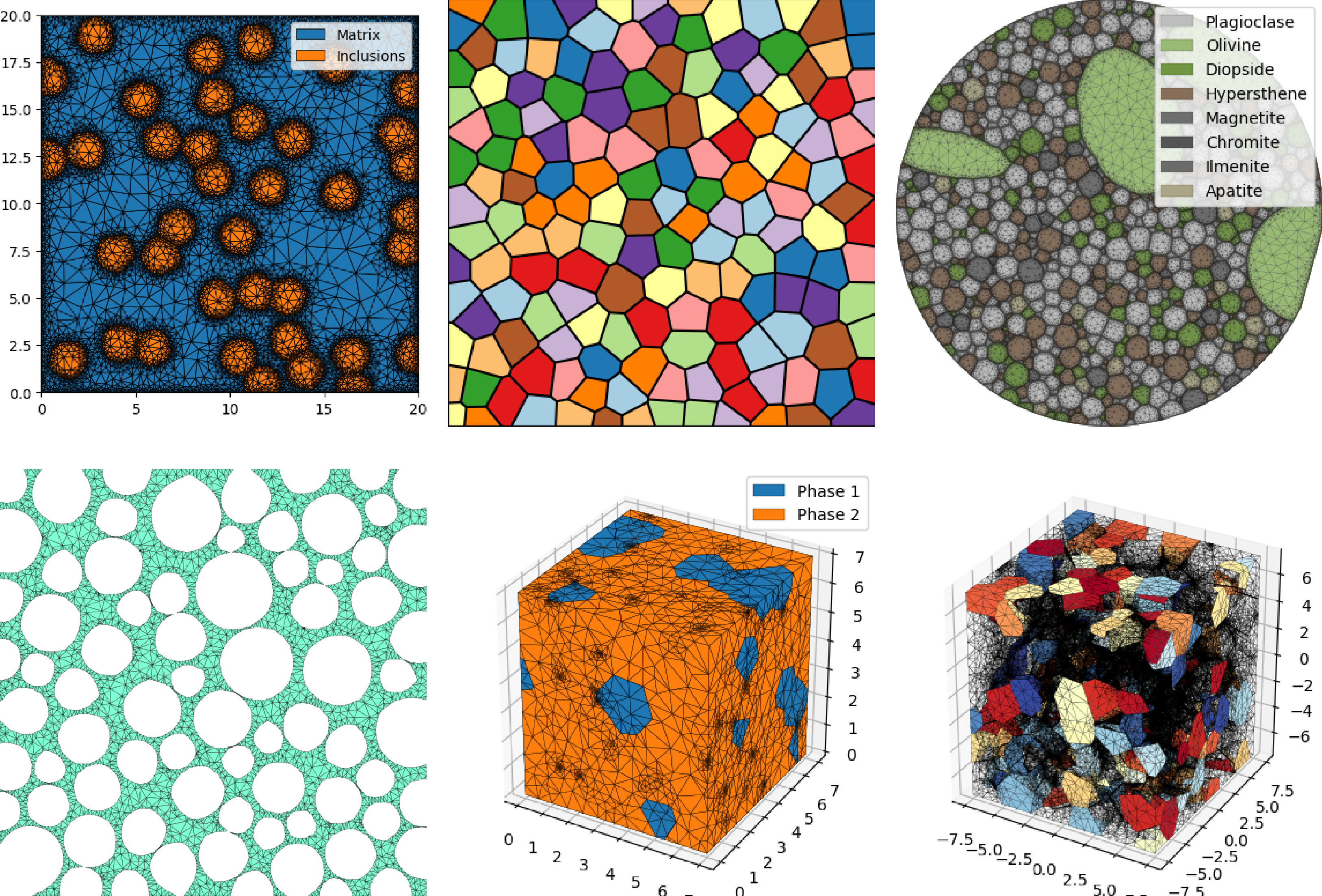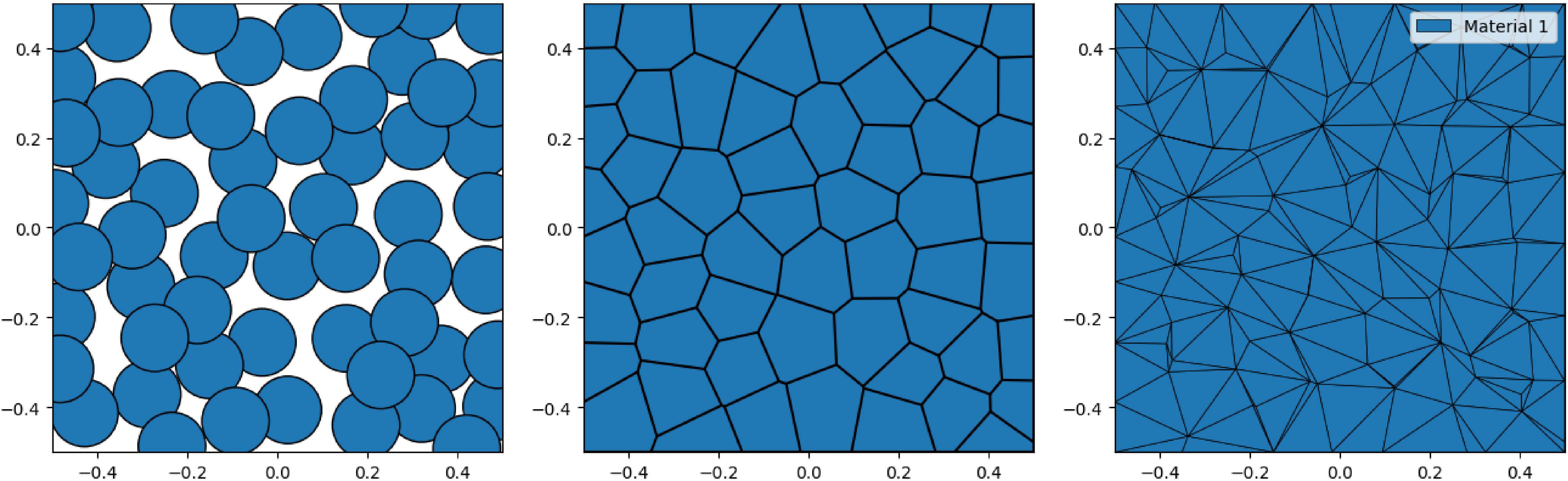# MicroStructPy - Microstructure Mesh Generation in Python¶RepositoryDocumentationPyPI

MicroStructPy is a microstructure mesh generator written in Python. Features of MicroStructPy include:

• 2D and 3D microstructures

• Grain size, shape, orientation, and position control

• Polycrystals, amorphous phases, and voids

• Mesh verification

• Visualizations

• Output to common file formats

• Customizable workflowFig. 1 The primary steps to create a microstructure. 1) seed the domain with particles, 2) create a Voronoi power diagram, and 3) convert the diagram into an unstructured mesh.

## Examples¶

These images were created using MicroStructPy. For more examples, see the Examples section.Fig. 2 Examples created using MicroStructPy.

## Quick Start¶

pip install microstructpy


If there is an error with the install, try pip install pybind11 first, then install MicroStructPy. This will create a command line executable and python package both named microstructpy. To use the command line interface, create a file called input.xml and copy this into it:

<?xml version="1.0" encoding="UTF-8"?>
<input>
<material>
<shape> circle </shape>
<size> 0.15 </size>
</material>

<domain>
<shape> square </shape>
</domain>
</input>


Next, run the file from the command line:

microstructpy input.xml


This will produce three text files and three image files: seeds.txt, polymesh.txt, trimesh.txt, seeds.png, polymesh.png, and trimesh.png. The text files contain all of the data related to the seed geometries and meshes. The image files contain:Fig. 3 The output plots are: 1) seed geometries, 2) polygonal mesh, and 3) triangular mesh.

The same results can be produced using this script:

import matplotlib.pyplot as plt
import microstructpy as msp

phase = {'shape': 'circle', 'size': 0.15}
domain = msp.geometry.Square()

# Unpositioned list of seeds
seeds = msp.seeding.SeedList.from_info(phase, domain.area)

# Position seeds in domain
seeds.position(domain)

# Create polygonal mesh
polygon_mesh = msp.meshing.PolyMesh.from_seeds(seeds, domain)

# Create triangular mesh
triangle_mesh = msp.meshing.TriMesh.from_polymesh(polygon_mesh)

# Plot outputs
for output in [seeds, polygon_mesh, triangle_mesh]:
plt.figure()
output.plot(edgecolor='k')
plt.axis('image')
plt.axis([-0.5, 0.5, -0.5, 0.5])
plt.show()